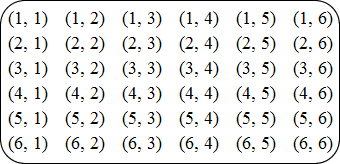index: click on a letter A B C D E F G H I J K L M N O P Q R S T U V W X Y Z A to Z index index: subject areas numbers & symbols sets, logic, proofs geometry algebra trigonometry advanced algebra & pre-calculus calculus advanced topics probability & statistics real world applications multimedia entrieswww.mathwords.com about mathwords website feedback

Sample Space

The set of all possible outcomes of an experiment.

 Example 1: A coin is flipped. Sample Space = {heads, tails} Example 2: Two six-sided dice are rolled. If (3, 5) means the first die shows 3 and the second die shows 5, then Sample Space =